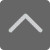TOP

深圳高中数学必修一函数的基本性质知识点总结归纳

2019-01-07 10:56:34 　来源：网络

函数的学习贯穿于整个高中数学，占有及其重要的位置。函数的基本性质是函数的基本主脉，是函数的知识主线，因此，我们要整体把握函数基本性质的基础知识、基本技能，在头脑中要有一个有效的知识网络，以下是小编为帮助大家更深入的了解初中数学知识所整理的深圳高中数学必修一函数的基本性质知识点总结归纳学习资料，一起来学习下吧!深圳高中数学必修一函数的基本性质知识点总结归纳

1奇偶性

(1)定义：如果对于函数f(x)定义域内的任意x都有f(-x)=-f(x)，则称f(x)为奇函数;如果对于函数f(x)定义域内的任意x都有f(-x)=f(x)，则称f(x)为偶函数。

如果函数f(x)不具有上述性质，则f(x)不具有奇偶性.如果函数同时具有上述两条性质，则f(x)既是奇函数，又是偶函数。

注意

1 函数是奇函数或是偶函数称为函数的奇偶性，函数的奇偶性是函数的整体性质;

2 由函数的奇偶性定义可知，函数具有奇偶性的一个必要条件是，对于定义域内的任意一个x，则-x也一定是定义域内的一个自变量(即定义域关于原点对称)。

(2)利用定义判断函数奇偶性的格式步骤：

1 首先确定函数的定义域并判断其定义域是否关于原点对称;

2 确定f(-x)与f(x)的关系;

3 作出相应结论：

若f(-x) = f(x) 或 f(-x)-f(x) = 0，则f(x)是偶函数;

若f(-x) =-f(x) 或 f(-x)+f(x) = 0，则f(x)是奇函数

(3)简单性质：

①图象的对称性质：一个函数是奇函数的充要条件是它的图象关于原点对称;一个函数是偶函数的充要条件是它的图象关于y轴对称;

②设 f(x)，g(x)的定义域分别是D1 ，D2，那么在它们的公共定义域上：

奇+奇=奇，奇 奇=偶，偶+偶=偶，偶 偶=偶，奇 偶=奇

2单调性

(1)定义：一般地，设函数y=f(x)的定义域为I，如果对于定义域I内的某个区间D内的任意两个自变量x1，x2，当x1f(x2))，那么就说f(x)在区间D上是增函数(减函数);

注意

1 函数的单调性是在定义域内的某个区间上的性质，是函数的局部性质;

2 必须是对于区间D内的任意两个自变量x1，x2;当x1

(2)如果函数y=f(x)在某个区间上是增函数或是减函数，那么就说函数y=f(x)在这一区间具有(严格的)单调性，区间D叫做y=f(x)的单调区间。

(3)设复合函数y= f[g(x)]，其中u=g(x) , A是y= f[g(x)]定义域的某个区间，B是映射g : x→u=g(x) 的象集：

①若u=g(x) 在 A上是增(或减)函数，y= f(u)在B上也是增(或减)函数，则函数y= f[g(x)]在A上是增函数;

②若u=g(x)在A上是增(或减)函数，而y= f(u)在B上是减(或增)函数，则函数y= f[g(x)]在A上是减函数。

(4)判断函数单调性的方法步骤

利用定义证明函数f(x)在给定的区间D上的单调性的一般步骤：

1 任取x1，x2∈D，且x1

2 作差f(x1)-f(x2);

3 变形(通常是因式分解和配方);

4 定号(即判断差f(x1)-f(x2)的正负);

5 下结论(即指出函数f(x)在给定的区间D上的单调性)。

(5)简单性质

①奇函数在其对称区间上的单调性相同;

②偶函数在其对称区间上的单调性相反;

③在公共定义域内：

增函数 增函数 是增函数;

减函数 减函数 是减函数;

增函数 减函数 是增函数;

减函数 增函数 是减函数。

3最值

(1)定义：

最大值：一般地，设函数y=f(x)的定义域为I，如果存在实数M满足：①对于任意的x∈I，都有f(x)≤M;②存在x0∈I，使得f(x0) = M。那么，称M是函数y=f(x)的最大值。

最小值：一般地，设函数y=f(x)的定义域为I，如果存在实数M满足：①对于任意的x∈I，都有f(x)≥M;②存在x0∈I，使得f(x0) = M。那么，称M是函数y=f(x)的最大值。

注意

1 函数最大(小)首先应该是某一个函数值，即存在x0∈I，使得f(x0) = M;

2 函数最大(小)应该是所有函数值中最大(小)的，即对于任意的x∈I，都有f(x)≤M(f(x)≥M)。

(2)利用函数单调性的判断函数的最大(小)值的方法：

1 利用二次函数的性质(配方法)求函数的最大(小)值;

2 利用图象求函数的最大(小)值;

3 利用函数单调性的判断函数的最大(小)值：如果函数y=f(x)在区间[a，b]上单调递增，在区间[b，c]上单调递减则函数y=f(x)在x=b处有最大值f(b);

如果函数y=f(x)在区间[a，b]上单调递减，在区间[b，c]上单调递增则函数y=f(x)在x=b处有最小值f(b);

所以我们在平常中要强调函数的单调性、奇偶性、周期性和最值的学习，并要熟悉基本初等函数的这些性质，通过练习提升自我，除了以上小编分享的深圳高中数学必修一函数的基本性质知识点总结归纳之外，如果同学们还想了解更多有关数学的其他学习内容，欢迎前往深圳学而思1对1官网进行查阅学习。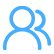请加入QQ群，试题资料免费放送！
 深圳小学交流群：254317299 一键加群 深圳初中交流群：90482695 一键加群 高一年级交流群：293049985 一键加群 高中交流群：175743089 一键加群 高二年级交流群：148082199 一键加群 2021高考交流群：644059693 一键加群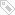标签：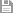保存　｜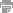打印　｜关闭